# Examples On Geometrical Interpretation Of Complex Equations

Go back to  'Complex Numbers'

Example - 19

Plot the region/locus represented by $$z$$ if $$z$$ satisfies:

(a)  $$\left| {z - 1} \right| = \left| {z + 1} \right|$$

(b)  $$\left| {z - 1 + i} \right| = \left| {z + 1 - i} \right|$$

(c)  $$2 < {\mathop{\rm Re}\nolimits} (z) < 3;\,\,\,2 < {\mathop{\rm Im}\nolimits} (z) < 3$$

(d) $$\left| {z - i} \right| + \left| {z + i} \right| = 3$$

Solution: (a) As mentioned earlier, we must look for a  geometrical  interpretations of equations involving complex numbers. This equation, in particular, says that the distance of $$z$$ from 1 (you must learn to view every number as a point on the complex plane; for example, 1 is a point which lies on the real axis!) must be equal to the distance of $$z$$ from –1, because

$\left| {z - 1} \right| = \left| {z - ( - 1)} \right|$

From plane geometry, $$z$$ must lie on the perpendicular bisector of 1 and –1, or equivalently $$z$$ must lie anywhere on the imaginary axis.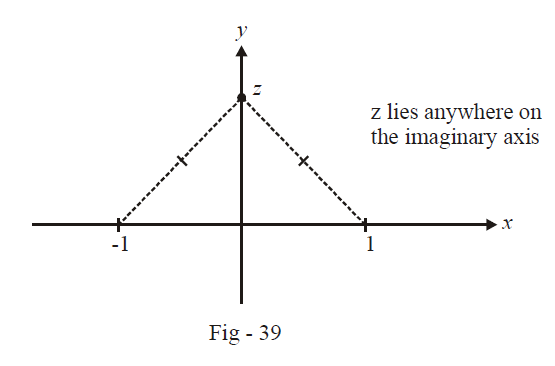(b)          As in part (a), this equation, which can be written as

$\left| {z - (1 - i)} \right| = \left| {z - ( - 1 + i)} \right|$

means that $$z$$ is equidistant from $$1–i$$ and $$–1+i,$$ i.e., $$z$$ lies on the perpendicular bisector of these two points: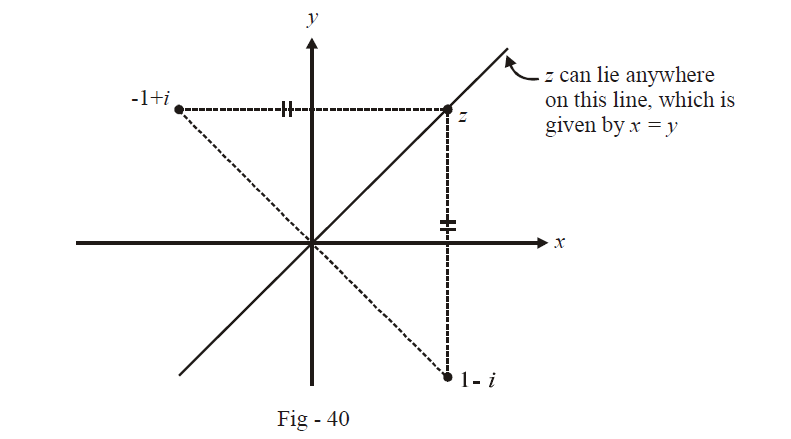(c) Since $$2 < {\mathop{\rm Re}\nolimits} \left( z \right) < 3,$$ $$z$$ lies anywhere in the region between the vertical lines $$x = 2$$ and $$x = 3.$$  Also, since $$2 < {\mathop{\rm Im}\nolimits} \left( z \right) < 3,\,\,\,z$$ must also lie in the region between the horizontal lines $$y = 2$$ and $$y = 3.$$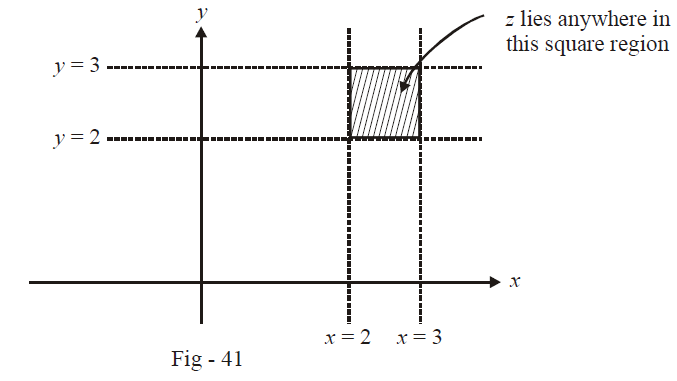(d) To solve this part, a little knowledge of co-ordinate geometry would be helpful. The given equation says that the sum of the distances of $$z$$ from $$i$$ and from $$–i$$ must equal 3. $$z$$ would therefore trace out an elliptical path in the plane with $$i$$ and $$–i$$ as its two foci, as shown in the figure below: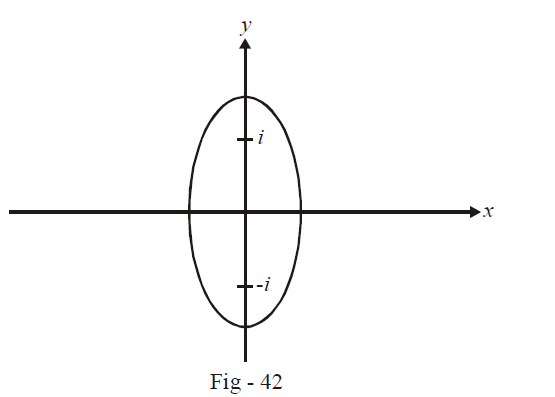For those not conversant with co-ordinate geometry, here’s an explanation. Suppose that you fix two pegs at the points $$i$$ and $$–i$$ and tie a 3-unit long string between the two pegs.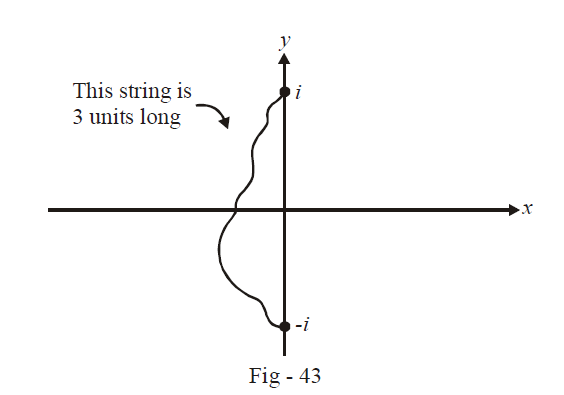Now, with a pen, pull this string to “away” from the pegs so that it becomes taut, and then, keeping the string taut, trace out a complete revolution on the plane with the tip of the pen (the taut string will automatically guide the pen)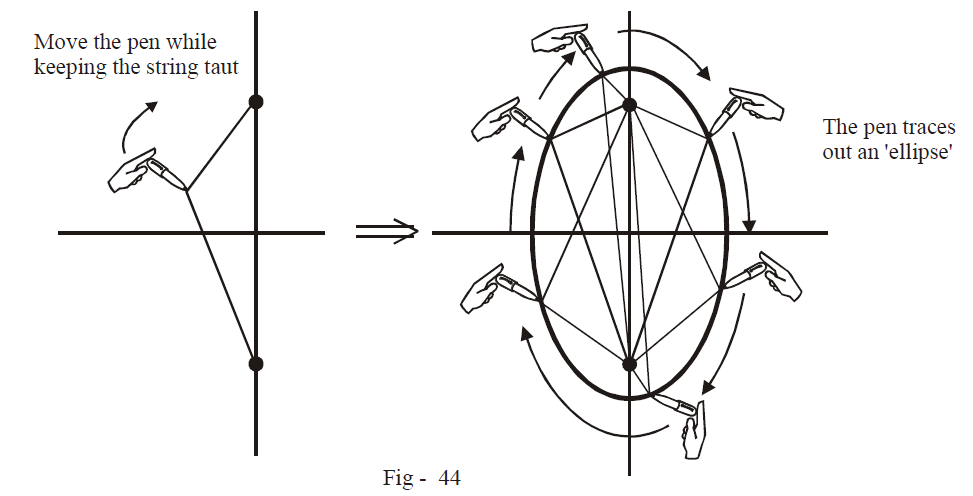Example - 20

Plot the locus of $$z$$ if $$z$$ satisfies \begin{align}\left| {\arg \left( {\frac{{z - 1}}{{z + 1}}} \right)} \right| = \frac{\pi }{2}\end{align}

Solution: We have,

\begin{align}{}&\arg \left( {\frac{{z - 1}}{{z + 1}}} \right) = \pm \frac{\pi }{2}\\ \Rightarrow \qquad &\arg \left( {z - 1} \right) - \arg \left( {z + 1} \right) = \pm \frac{\pi }{2}\,\,\,\,\,\,\,\,\,\,\,\,\,\,\,\,\,\,\,\,\,\,\,\,\,\,\,\,...{\rm{ }}\left( 1 \right)\end{align}

$$z – 1$$ is the vector drawn from the point 1 to the point $$z.$$ Similarly, $$z + 1$$ is the Vectron from –1 to $$z.$$ The angle between these two vectors, as (1) tells us, is\begin{align} \pm \frac{\pi }{2}\end{align}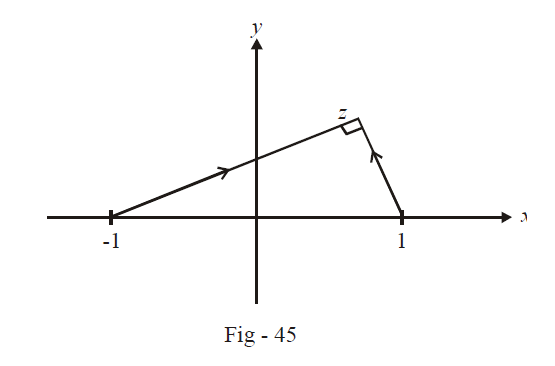Since the angle in a semicircle is a right angle, $$z$$ can lie anywhere on a circle with 1 and –1 as the  end-points of a diameter. (1 and –1 themselves cannot lie on this circle because either $$z – 1$$ or $$z + 1$$ becomes a zero vector if $$z = 1$$ or $$–1,$$ and the argument of a zero vector cannot be uniquely defined)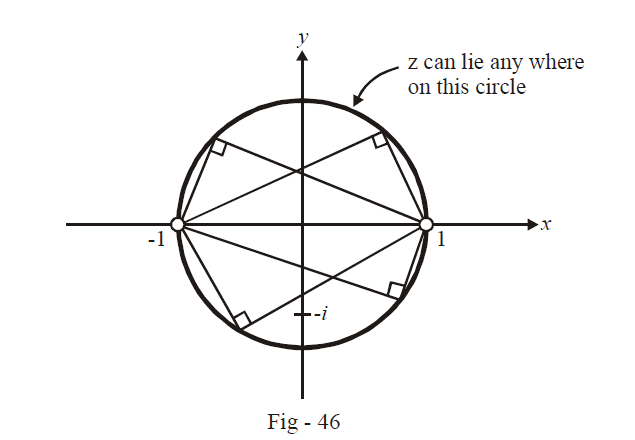## TRY YOURSELF - V

Q. 1 Plot the locus / region represented by $$z$$ in the following cases:

 (a) $$|z|\; \in \;[1,\;2]\; \cup [3,\;4]$$ (b) \begin{align}|z|\; < 3,\;\frac{\pi }{6} < \;{\rm{Arg}}(z) < \frac{\pi }{3}\end{align} (c) \begin{align}1\, < \,|z|\; < \,2,\;\frac{\pi }{6}\; < \;|{\rm{Arg}}(z)|\; < \;\frac{\pi }{3}\end{align} (d) $$|z|\; < 2,\;1 < \;|{\mathop{\rm Re}\nolimits} (z)|\; < 2,\;1 < \;|{\mathop{\rm Im}\nolimits} (z)|\; < 2$$ (e) $$|z - 1{|^2} + |z + 1{|^2} = 4$$ (f) $$|z - i{|^2} + |z + i{|^2} = 4$$ (g) $$|z - 2 + 2i|\; = \;|z + 2 + 2i|$$ (h) $${\mathop{\rm Re}\nolimits} (z) + {\mathop{\rm Im}\nolimits} (z) = 1$$ (i) $$|{\mathop{\rm Re}\nolimits} (z)| + |{\mathop{\rm Im}\nolimits} (z)|\; \le 1$$ (j) $$|z - 2|\; + \;|z + 2i|\; = 5$$ (k) \begin{align}\arg \left( {\frac{{z - 1}}{{z + 1}}} \right) = \frac{\pi }{6}\end{align} (l) \begin{align}|z|\; < \;3,\;|z - 4i|\; < \;2,\;\;\arg (z) < \frac{\pi }{2}\end{align} (m) $$\sin |z|\; > 0$$ (n) $$|z|\; < \;1,\;\;\tan (\arg (z))\; > 1$$ (o) $$|z{|^3} - 6|z{|^2} + 11|z| - 6 \ge 0,$$ $$|z{|^2} - 5|z| + \;6 \le 0$$
Learn from the best math teachers and top your exams

• Live one on one classroom and doubt clearing
• Practice worksheets in and after class for conceptual clarity
• Personalized curriculum to keep up with school